# Modeling a Family of Responses as an Uncertain System

This example shows how to use the Robust Control Toolbox™ command `ucover` to model a family of LTI responses as an uncertain system. This command is useful to fit an uncertain model to a set of frequency responses representative of the system variability, or to reduce the complexity of an existing uncertain model to facilitate the synthesis of robust controllers with `musyn`.

### Modeling Plant Variability as Uncertainty

In this first example, we have a family of models describing the plant behavior under various operating conditions. The nominal plant model is a first-order unstable system.

```Pnom = tf(2,[1 -2]) ```
```Pnom = 2 ----- s - 2 Continuous-time transfer function. ```

The other models are variations of `Pnom`. They all have a single unstable pole but the location of this pole may vary with the operating condition.

```p1 = Pnom*tf(1,[.06 1]); % extra lag p2 = Pnom*tf([-.02 1],[.02 1]); % time delay p3 = Pnom*tf(50^2,[1 2*.1*50 50^2]); % high frequency resonance p4 = Pnom*tf(70^2,[1 2*.2*70 70^2]); % high frequency resonance p5 = tf(2.4,[1 -2.2]); % pole/gain migration p6 = tf(1.6,[1 -1.8]); % pole/gain migration ```

To apply robust control tools, we can replace this set of models with a single uncertain plant model whose range of behaviors includes `p1` through `p6`. This is one use of the command `ucover`. This command takes an array of LTI models `Parray` and a nominal model `Pnom` and models the difference `Parray-Pnom` as multiplicative uncertainty in the system dynamics.

Because `ucover` expects an array of models, use the `stack` command to gather the plant models `p1` through `p6` into one array.

```Parray = stack(1,p1,p2,p3,p4,p5,p6); ```

Next, use `ucover` to "cover" the range of behaviors `Parray` with an uncertain model of the form

`P = Pnom * (1 + Wt * Delta)`

where all uncertainty is concentrated in the "unmodeled dynamics" `Delta` (a `ultidyn` object). Because the gain of `Delta` is uniformly bounded by 1 at all frequencies, a "shaping" filter `Wt` is used to capture how the relative amount of uncertainty varies with frequency. This filter is also referred to as the uncertainty weighting function.

Try a 4th-order filter `Wt` for this example:

```orderWt = 4; Parrayg = frd(Parray,logspace(-1,3,60)); [P,Info] = ucover(Parrayg,Pnom,orderWt,'InputMult'); ```

The resulting model `P` is a single-input, single-output uncertain state-space (USS) object with nominal value `Pnom`.

```P ```
```P = Uncertain continuous-time state-space model with 1 outputs, 1 inputs, 5 states. The model uncertainty consists of the following blocks: Parrayg_InputMultDelta: Uncertain 1x1 LTI, peak gain = 1, 1 occurrences Type "P.NominalValue" to see the nominal value, "get(P)" to see all properties, and "P.Uncertainty" to interact with the uncertain elements. ```
```tf(P.NominalValue) ```
```ans = 2 ----- s - 2 Continuous-time transfer function. ```

A Bode magnitude plot confirms that the shaping filter `Wt` "covers" the relative variation in plant behavior. As a function of frequency, the uncertainty level is 30% at 5 rad/sec (-10dB = 0.3) , 50% at 10 rad/sec, and 100% beyond 29 rad/sec.

```Wt = Info.W1; bodemag((Pnom-Parray)/Pnom,'b--',Wt,'r'); grid title('Relative Gaps vs. Magnitude of Wt') ```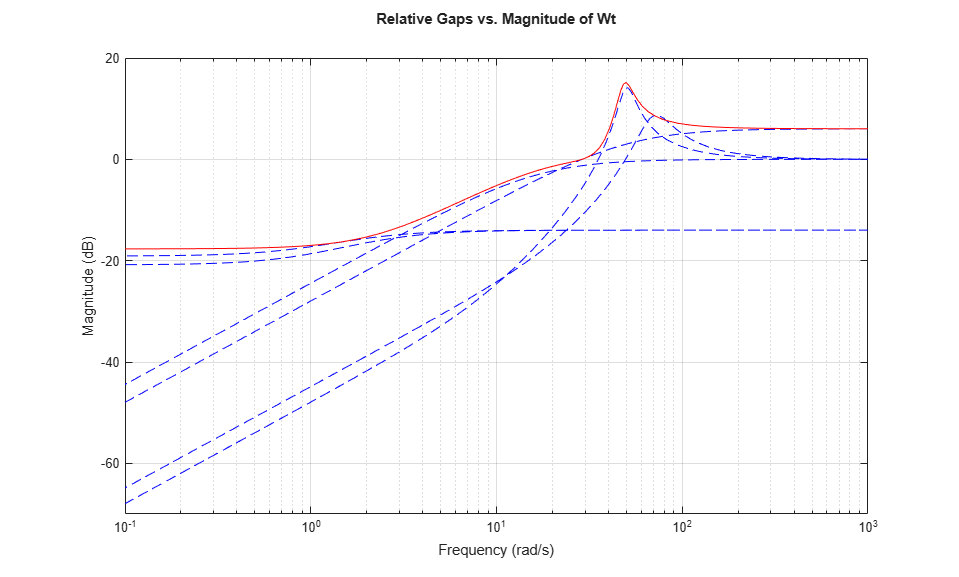You can now use the uncertain model `P` to design a robust controller for the original family of plant models, see Simultaneous Stabilization Using Robust Control for details.

### Simplifying an Existing Uncertain Model

In this second example, we start with a detailed uncertain model of the plant. This model consists of first-order dynamics with uncertain gain and time constant, in series with a mildly underdamped resonance and significant unmodeled dynamics. This model is created using the `ureal` and `ultidyn` commands for specifying uncertain variables:

```gamma = ureal('gamma',2,'Perc',30); % uncertain gain tau = ureal('tau',1,'Perc',30); % uncertain time-constant wn = 50; xi = 0.25; P = tf(gamma,[tau 1]) * tf(wn^2,[1 2*xi*wn wn^2]); % Add unmodeled dynamics and set SampleStateDim to 5 to get representative % sample values of the uncertain model P delta = ultidyn('delta',[1 1],'SampleStateDim',5,'Bound',1); W = makeweight(0.1,20,10); P = P * (1+W*delta) ```
```P = Uncertain continuous-time state-space model with 1 outputs, 1 inputs, 4 states. The model uncertainty consists of the following blocks: delta: Uncertain 1x1 LTI, peak gain = 1, 1 occurrences gamma: Uncertain real, nominal = 2, variability = [-30,30]%, 1 occurrences tau: Uncertain real, nominal = 1, variability = [-30,30]%, 1 occurrences Type "P.NominalValue" to see the nominal value, "get(P)" to see all properties, and "P.Uncertainty" to interact with the uncertain elements. ```

A collection of step responses illustrates the plant variability.

```step(P,4) title('Sampled Step Responses of Uncertain System') ```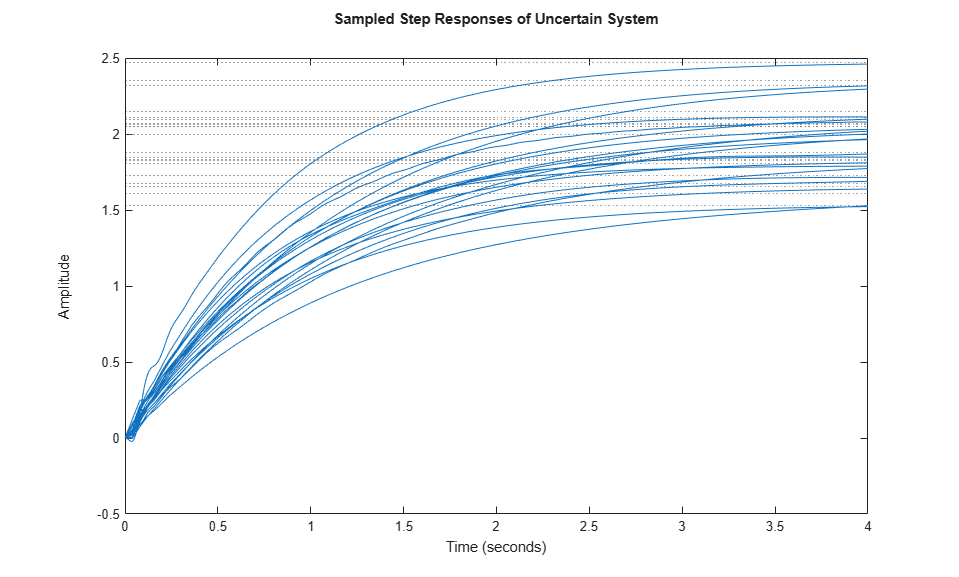The uncertain plant model `P` contains 3 uncertain elements. For control design purposes, it is often desirable to simplify this uncertainty model while approximately retaining its overall variability. This is another use of the command `ucover`.

To use `ucover` in this context, first map the uncertain model `P` into an array of LTI models using `usample`. This command samples the uncertain elements in an uncertain system and returns the corresponding LTI models, each model representing one possible behavior of the uncertain system. In this example, sample `P` at 60 points (the random number generator is seeded for repeatability):

```rng(0,'twister'); Parray = usample(P,60); ```

Next, use `ucover` to cover all behaviors in `Parray` by a simple uncertainty model `Usys`. Choose the nominal value of `P` as center of the cover, and use a 2nd-order filter to model the frequency distribution of the unmodeled dynamics.

```orderWt = 2; Parrayg = frd(Parray,logspace(-3,3,60)); [Usys,Info] = ucover(Parrayg,P.NominalValue,orderWt,'InputMult'); ```

A Bode magnitude plot shows how the filter magnitude (in red) "covers" the relative variability of the plant frequency response (in blue).

```Wt = Info.W1; bodemag((P.NominalValue-Parray)/P.NominalValue,'b--',Wt,'r') title('Relative Gaps (blue) vs. Shaping Filter Magnitude (red)') ```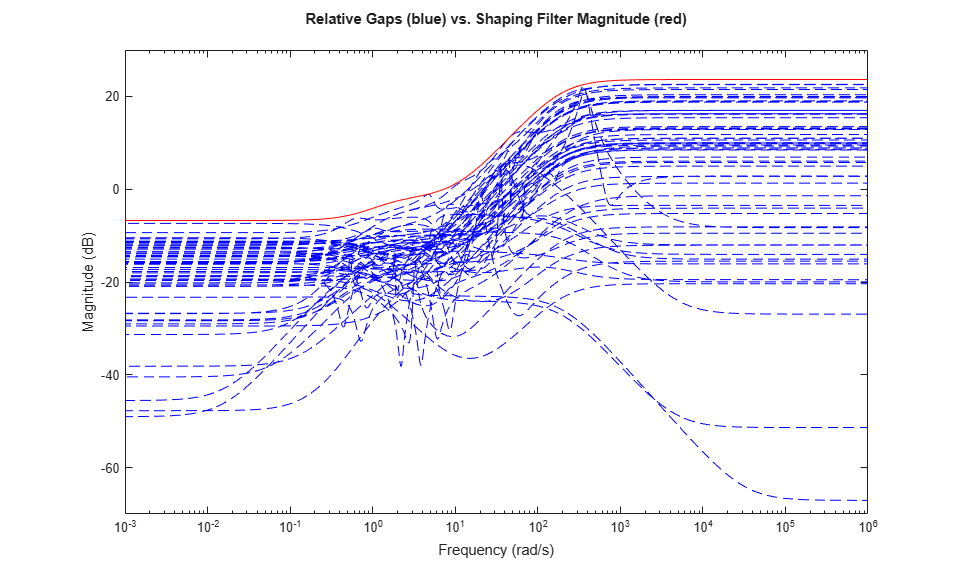You can now use the simplified uncertainty model `Usys` to design a robust controller for the original plant, see First-Cut Robust Design for details.

In this third example, we start with 40 frequency responses of a 2-input, 2-output system. This data has been collected with a frequency analyzer under various operating conditions. A two-state nominal model is fitted to the most typical response:

```A = [-5 10;-10 -5]; B = [1 0;0 1]; C = [1 10;-10 1]; D = 0; Pnom = ss(A,B,C,D); ```

The frequency response data is loaded into a 40-by-1 array of FRD models:

```load ucover_demo size(Pdata) ```
```40x1 array of FRD models. Each model has 2 outputs, 2 inputs, and 120 frequency points. ```

Plot this data and superimpose the nominal model.

```bode(Pdata,'b--',Pnom,'r',{.1,1e3}), grid legend('Frequency response data','Nominal model','Location','NorthEast') ```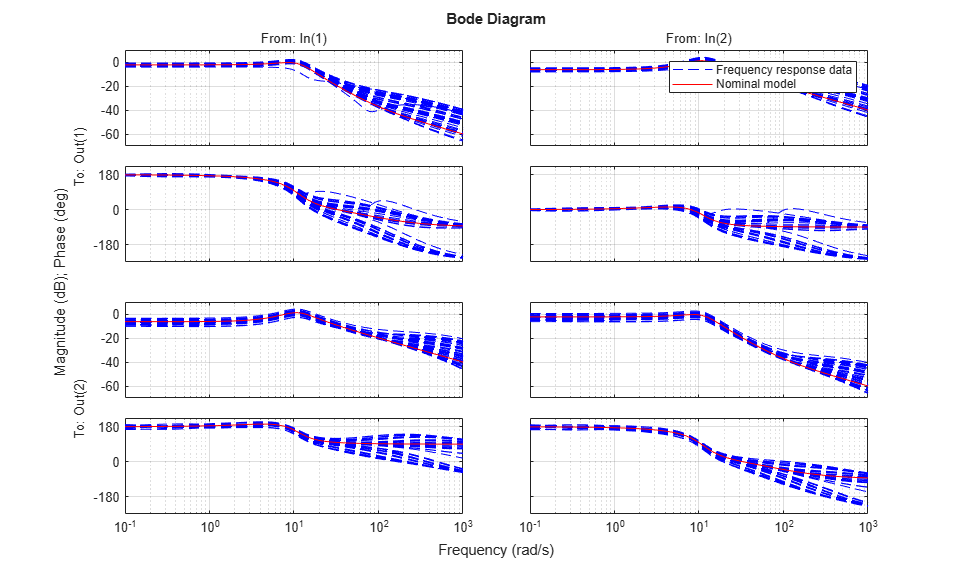Because the response variability is modest, try modeling this family of frequency responses using an additive uncertainty model of the form

`P = Pnom + w * Delta`

where `Delta` is a 2-by-2 `ultidyn` object representing the unmodeled dynamics and `w` is a scalar weighting function reflecting the frequency distribution of the uncertainty (variability in Pdata).

Start with a first-order filter `w` and compare the magnitude of `w` with the minimum amount of uncertainty needed at each frequency:

```[P1,InfoS1] = ucover(Pdata,Pnom,1,'Additive'); w = InfoS1.W1; bodemag(w,'r',InfoS1.W1opt,'g',{1e-1 1e3}) title('Scalar Additive Uncertainty Model') legend('First-order w','Min. uncertainty amount','Location','SouthWest') ```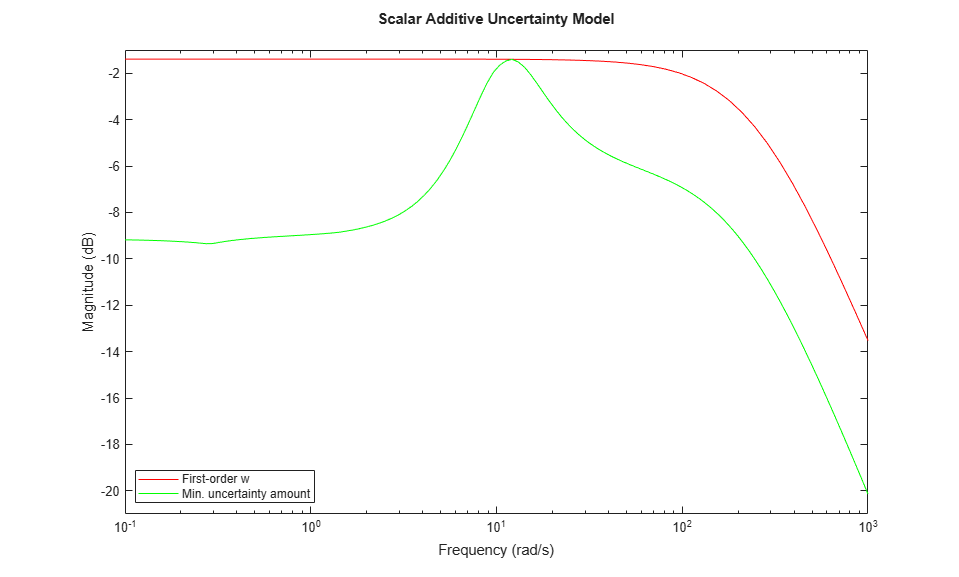The magnitude of `w` should closely match the minimum uncertainty amount. It is clear that the first-order fit is too conservative and exceeds this minimum amount at most frequencies. Try again with a third-order filter `w`. For speed, reuse the data in `InfoS1` to avoid recomputing the optimal uncertainty scaling at each frequency.

```[P3,InfoS3] = ucover(Pnom,InfoS1,3,'Additive'); w = InfoS3.W1; bodemag(w,'r',InfoS3.W1opt,'g',{1e-1 1e3}) title('Scalar Additive Uncertainty Model') legend('Third-order w','Min. uncertainty amount','Location','SouthWest') ```The magnitude of `w` now closely matches the minimum uncertainty amount. Among additive uncertainty models, `P3` provides a tight cover of the behaviors in `Pdata`. Note that `P3` has a total of 8 states (2 from the nominal part and 6 from `w`).

```P3 ```
```P3 = Uncertain continuous-time state-space model with 2 outputs, 2 inputs, 8 states. The model uncertainty consists of the following blocks: Pdata_AddDelta: Uncertain 2x2 LTI, peak gain = 1, 1 occurrences Type "P3.NominalValue" to see the nominal value, "get(P3)" to see all properties, and "P3.Uncertainty" to interact with the uncertain elements. ```

You can refine this additive uncertainty model by using non-scalar uncertainty weighting functions, for example

```P = Pnom + W1*Delta*W2 ```

where `W1` and `W2` are 2-by-2 diagonal filters. In this example, restrict use `W2=1` and allow both diagonal entries of W1 to be third order.

```[PM,InfoM] = ucover(Pdata,Pnom,[3;3],[],'Additive'); ```

Compare the two entries of `W1` with the minimum uncertainty amount computed earlier. Note that at all frequencies, one of the diagonal entries of `W1` has magnitude much smaller than the scalar filter `w`. This suggests that the diagonally-weighted uncertainty model yields a less conservative cover of the frequency response family.

```bodemag(InfoS1.W1opt,'g*',... InfoM.W1opt(1,1),'r--',InfoM.W1(1,1),'r',... InfoM.W1opt(2,2),'b--',InfoM.W1(2,2),'b',{1e-1 1e3}); title('Diagonal Additive Uncertainty Model') legend('Scalar Optimal Weight',... 'W1(1,1), pointwise optimal',... 'W1(1,1), 3rd-order fit',... 'W1(2,2), pointwise optimal',... 'W1(2,2), 3rd-order fit',... 'Location','SouthWest') ```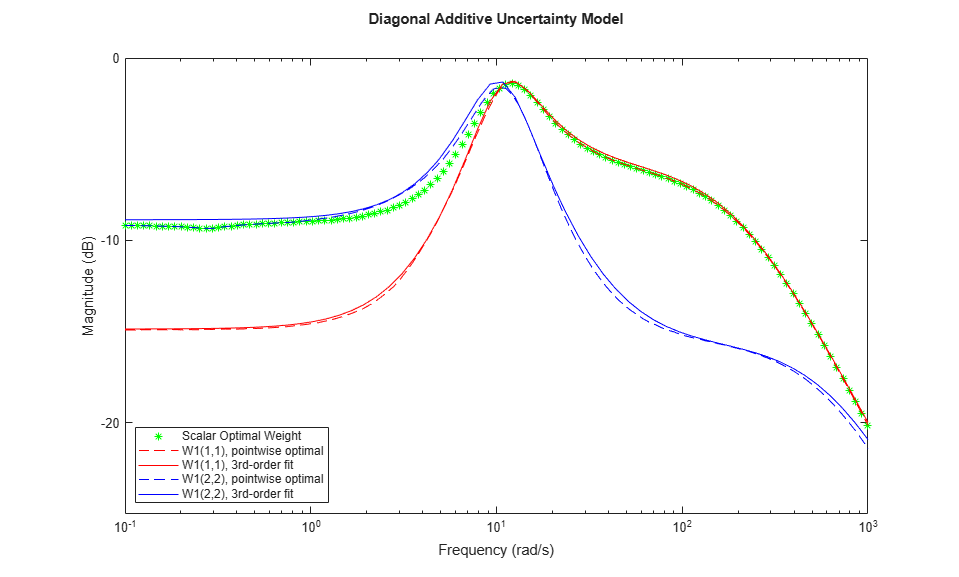The degree of conservativeness of one cover over another can be partially quantified by considering the two frequency-dependent quantities:

`Fd2s = norm(inv(W1)*w) , Fs2d = norm(W1/w)`

These quantities measure by how much one uncertainty model needs to be scaled to cover the other. For example, the uncertainty model `Pnom + W1*Delta` needs to be enlarged by a factor `Fd2s` to include all of the models represented by the uncertain model `Pnom + w*Delta`.

Plot `Fd2s` and `Fs2d` as a function of frequency.

```Fd2s = fnorm(InfoS1.W1opt*inv(InfoM.W1opt)); Fs2d = fnorm(InfoM.W1opt*inv(InfoS1.W1opt)); semilogx(fnorm(Fd2s),'b',fnorm(Fs2d),'r'), grid axis([0.1 1000 0.5 2.6]) xlabel('Frequency (rad/s)'), ylabel('Magnitude') title('Scale factors relating different covers') legend('Diagonal to Scalar factor',... 'Scalar to Diagonal factor','Location','SouthWest'); ```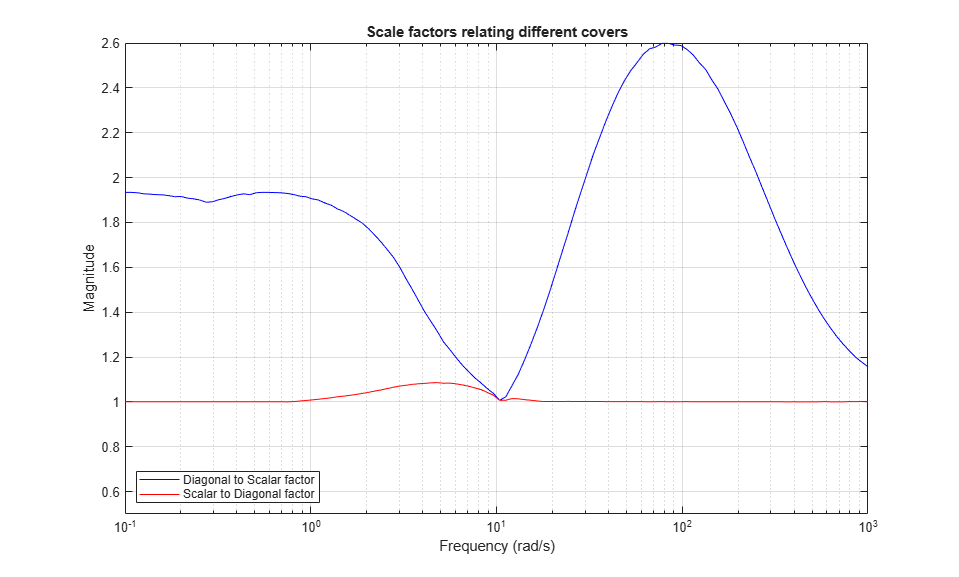This plot shows that:

• `Fs2d = 1` in a large frequency range so `Pnom+w*Delta` includes all the behaviors modeled by `Pnom+W1*Delta`

• In that same frequency range, `Pnom+W1*Delta` does not include all of the behaviors modeled by `Pnom+w*Delta` and, in fact, would need to be enlarged by a factor between 1.2 and 2.6 in order to do so.

• In the frequency range [1 20], neither uncertainty model contains the other, but at all frequencies, making `Pnom+W1*Delta` cover `Pnom+w*Delta` requires a much smaller scaling factor than the converse.

This indicates that the `Pnom+W1*Delta` model provides a less conservative cover of the frequency response data in `Pdata`.

##### SupportGet trial now